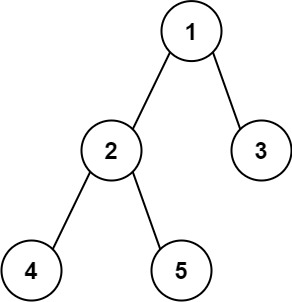# 543. Diameter of Binary Tree

Easy
Given the `root` of a binary tree, return the length of the diameter of the tree.
The diameter of a binary tree is the length of the longest path between any two nodes in a tree. This path may or may not pass through the `root`.
The length of a path between two nodes is represented by the number of edges between them.
Example 1:Input: root = [1,2,3,4,5]
Output: 3
Explanation: 3 is the length of the path [4,2,1,3] or [5,2,1,3].
Example 2:
Input: root = [1,2]
Output: 1
Constraints:
• The number of nodes in the tree is in the range `[1, 10^4]`.
• `-100 <= Node.val <= 100`

### 解題

/**
* Definition for a binary tree node.
* type TreeNode struct {
* Val int
* Left *TreeNode
* Right *TreeNode
* }
*/
func diameterOfBinaryTree(root *TreeNode) int {
ans := 0
if root == nil {
return ans
}
var helper func(*TreeNode) int
helper = func(root *TreeNode) int {
if root == nil {
return 0
}
ld := helper(root.Left)
rd := helper(root.Right)
ans = max(ans, ld + rd)
return max(ld, rd) + 1
}
helper(root)
return ans
}
func max(a, b int) int {
if a > b {
return a
}
return b
}